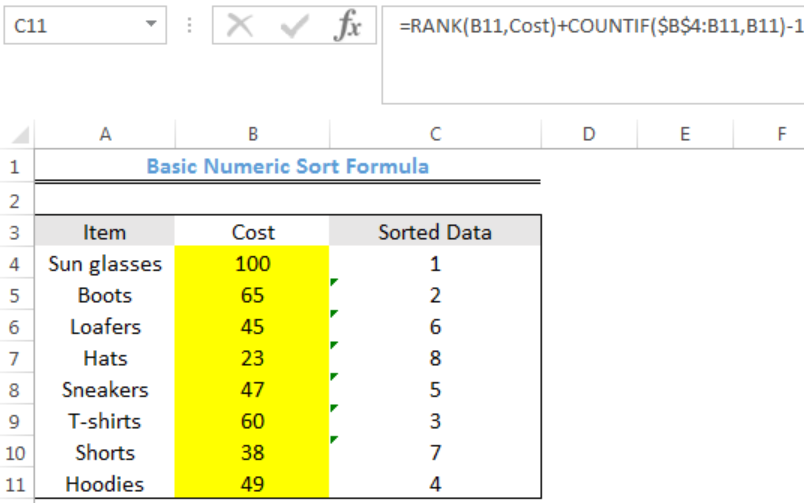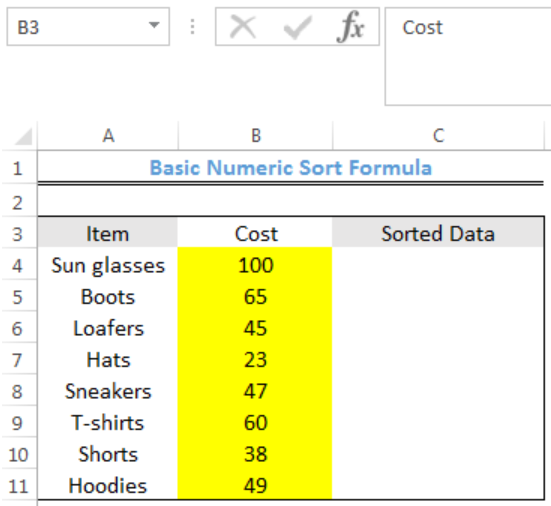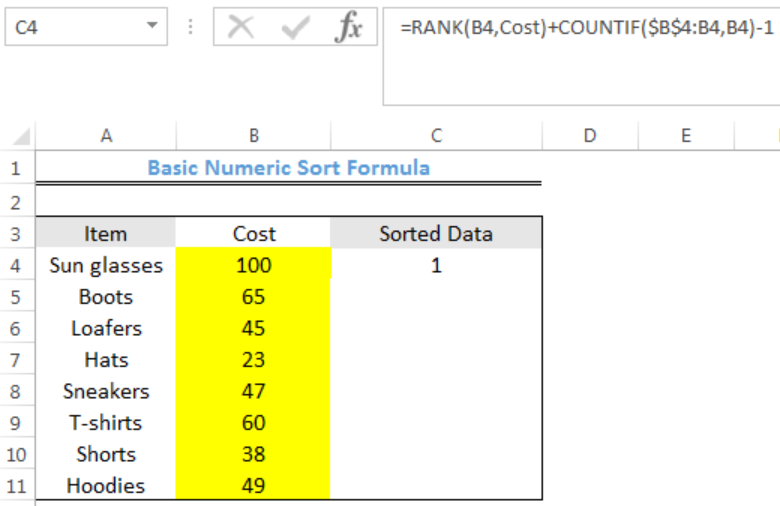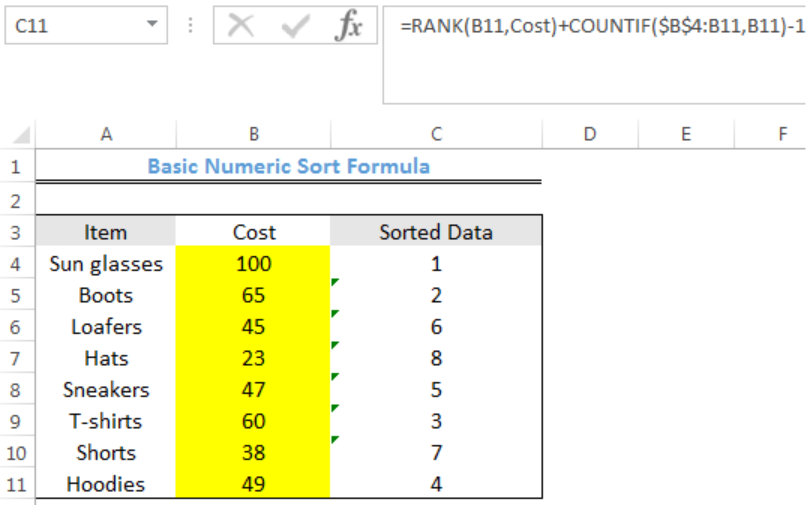Get instant live expert help with Excel or Google Sheets“My Excelchat expert helped me in less than 20 minutes, saving me what would have been 5 hours of work!”

#### Post your problem and you’ll get Expert help in seconds.

Your message must be at least 40 characters
Our professional Expert are available now. Your privacy is guaranteed.

# How to Use Basic Numeric Sort Formula

With a helper column, we can use a combination of the RANK and COUNTIF Functions to dynamically sort data that contains only numeric values. The steps below will walk through the process.Figure 1: Basic Numeric Sort Formula

## Syntax

`=COUNTIF(range,criteria)`

• COUNTIF count cells with numbers, dates and certain text that match specific criteria in a range.
`=RANK(number,ref[order])`
• RANK function is used to determine the position of a value in an array.

## Formula

`=RANK(B4,Cost)+COUNTIF(\$B\$4:B4,B4)-1`

## Setting up the Data

• We will set up the data by inputting the items into Column A
• We will input the cost of each item into Column B
• Column C is where we want the formula to return the result of the sorted data
• Note- We must highlight Cell B4 to B11 and name it as Cost by clicking on B3 where there is a drop-down arrow in figure 2Figure 2: Setting up the Data

## Numerical Sorting of the Data

• We will click on Cell C4
• We will insert the formula below into the cell
`=RANK(B4,Cost)+COUNTIF(\$B\$4:B4,B4)-1`
• We will press the enter keyFigure 3: Result for Cell B4

• We will click on Cell C4 again
• We will double-click on the fill handle (the small plus sign at the bottom right of Cell C4) and drag down to copy the formula into the other cellsFigure 4: Results of basic numeric sort formula

## Explanation

`=RANK(B4,Cost)+COUNTIF(\$B\$4:B4,B4)-1`

RANK uses the named range (Cost) to generate values from the highest to lowest starting with 1.

COUNTIF is required if there are duplicate values. A mixed reference is used to enter the range so that the formula continuously expands when copied to the other cells. We have to subtract 1 to zero out this effect. The formula, therefore, returns zero until a duplicate value is found and returns 1 at the second instance.

## Instant Connection to an Expert through our Excelchat Service

Most of the time, the problem you will need to solve will be more complex than a simple application of a formula or function. If you want to save hours of research and frustration, try our live Excelchat service! Our Excel Experts are available 24/7 to answer any Excel question you may have. We guarantee a connection within 30 seconds and a customized solution within 20 minutes.

### Did this post not answer your question? Get a solution from connecting with the expert.Another blog reader asked this question today on Excelchat:## Subscribe to Excelchat.coAnother blog reader asked this question today on Excelchat: